Refer to our Texas Go Math Grade 6 Answer Key Pdf to score good marks in the exams. Test yourself by practicing the problems from Texas Go Math Grade 6 Unit 7 Study Guide Review Answer Key.

Module 18 Becoming a Knowledgeable Consumer and Investor

Question 1.
Emily is looking for a bank. Compare the two banks in the table. Emily has an average balance of $500, writes 15 checks per month, and uses her debit card at an ATM 17 times per month. (Lesson 18.1)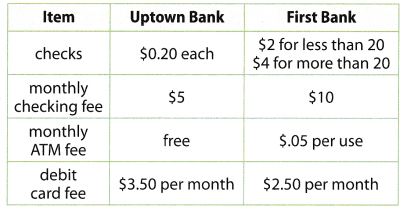a. Calculate her total monthly fee for each bank. Answer: Determine the monthly fee. Uptown Bank$0.20 × 15 = $3 check charges$3 + $5 +$3.50 = $11.50 monthly fee for Uptown Bank First Bank$0.05 × 17 = $0.85 monthly ATM fee$2 + $10$0.85 + $2.50 =$15.35 monthly fee for First Bank

b. Which is the best choice for her?
Based on the computation, Uptown Bank is best choice for Emily. As seen on the given situation, she always use her ATM in a month. Therefore it will be best if her bank gives free ATM fee.

Calculate the debt-to-income ratio for the following. (Lesson 18.2)

Question 2.
Damien has a monthly income of $2,500, a car payment of$400, and credit card payments of $100. __________________ Answer: Determine the debt-to-income ratio.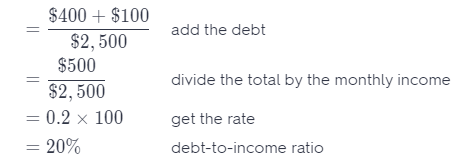The debt-to-income ratio is 20%. Question 3. Mara has a monthly income of$1,000, a credit card payment of $50, and a student loan payment of$75. _________________
Determine the debt-to-income ratio.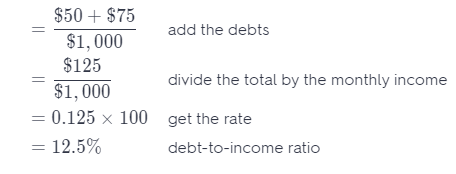The debt-to-income ratio is 12.5%.

Question 4.
Alicia has a monthly income of $4,000 and a student loan payment of$300. _____________
Determine the debt-to-income ratio.
= $$\frac{\ 300}{\ 4,000}$$ divide the loan by the monthly income
= 0.075 × 100 get the rate
= 7.5% debt-to-income ratio
The debt-to-income ratio is 7.5%.

Question 5.
Steven has a monthly income of $500 and a credit card payment of$25. ____________
Determine the debt-to-income ratio.
= $$\frac{\ 25}{\ 500}$$ divide the payment by the monthly income
= 0.05 × 100 get the rate
= 5% debt-to-income ratio
The debt-to-income ratio is 5%

Question 6.
Median income is $35,000 per year for a truck driver,$3,400 per month for a middle school teacher, and $450 per week for a bank teller. (Lesson 18.4) a. Which profession makes the most per year? Answer:$3,400 × 12 = $40,800 income of middle school teacher in a year$450 × 52 = $23,400 income of a bank teller in a year The middle school teacher makes the most per year compared to the truck driver and bank teller. b. Compare the incomes of a truck driver and a bank teller over 20 years. Show your work. Answer: Compare the incomes of a truck driver and a bank teller over 20 years.$35, 000 × 20 = $700,000 income in 20 years of a truck driver$23,400 × 20 = $468,000 income in 20 years of a bank teller$700,000 – $468,000 =$232,000 difference on the incomes
Middle school teacher earns the biggest than the other two professions per year.

c. Compare the income of a truck driver to a middle school teacher over 20 years. Show your work.
Compare the incomes of a truck driver and a middle school teacher over 20 years.
$35,000 × 20 =$700,000 income in 20 years of a truck driver
$40,800 × 20 =$816,000 income in 20 years of a middle school teacher
$816,000 –$700,000 = $116,000 difference on the incomes The middle school teacher earns$116,000 more than the truck driver

Question 1.
CAREERS IN MATH Bicycle Tour Operator Viktor is a bike tour operator and needs to replace two of his touring bikes. He orders two bikes from the sporting goods store for a total of $2,000 and pays using his credit card. When the bill arrives, he reads the following information: Balance:$2,000
Annual interest rate: 14.9%
Minimum payment due: $40 Late fee:$10 if payment not received by 3/1/2013

a. To keep his good credit, Viktor promptly sends in a minimum payment of $40. When the next bill arrives, it looks a lot like the previous bill. Balance:$1,984.34
Annual interest rate: 14.9%
Minimum payment due: $40 Late fee:$10 if payment not received by 4/1/2013
Explain how the credit card company calculated the new balance. Notice that the given interest rate is annual, but the payment is monthly.

b. Viktor was upset about the new bill, so he decided to send in $150 for his April payment. The minimum payment on his bill is calculated as 2% of the balance (rounded to the nearest dollar) or$20, whichever is greater. Fill out the details for Viktor’s new bill.
Balance:
Annual interest rate:
Minimum payment due:
Late fee: $10 if payment not received by Answer: c. Viktor’s bank offers a credit card with an introductory annual interest rate of 9.9%. He can transfer his current balance for a fee of$40. After one year, the rate will return to the bank’s normal rate, which is 13.9%. The bank charges a late fee of $15. Give two reasons why Viktor should transfer the balance, and two reasons why he should not. Answer: Question 2. Manuel has a monthly income of$440. Currently, his only monthly payment is his portion of the rent, which is $110. However, he is considering buying a used car. a. Calculate Manuel’s current debt-to-income ratio. Answer: Determine the current debt-to-income ratio. = $$\frac{\ 110}{\ 440}$$ divide the debt by the monthly income = 0.25 × 100 get the rate = 25% debt-to-income ratio b. If Manuel would like to maintain a debt-to-income ratio that is less than 60%, can he afford a monthly car payment of$175? Show your work.
Determine the debt-to-income ratio for the indicated payment.
= $$\frac{\ 175}{\ 440}$$ divide the debt by the monthly income
≈ 0.40 × 100 get the rate
≈ 40% debt-to-income ratio
Yes, he can afford a monthly payment of $175 because the debt-to-income ratio is less than 60% c. Manuel thinks he can maintain a debt-to-income ratio that is less than 60% if his car payment is less than$154. Is he correct? Explain.
Determine the debt-to-income ratio for the indicated payment.
= $$\frac{\ 154}{\ 440}$$ divide the debt by the monthly income
= 0.35 × 100 get the rate
= 35% debt-to-income ratio
Yes, he can afford a monthly payment of $154 because the debt-to-income ratio is less than 60%. Question 3. Lillian is a high school science teacher. She makes$50,230 per year and plans to teach for another 16 years. Lillian’s family is moving, so she will need to find a new school at which to teach. Lillian’s real estate agent said,”In the city you are moving to, salaries for teaching high school are 12% higher than the national average for a high school teacher.”
a. Lillian currently makes $3,000 less than the national average for a high school teacher. How much could she make in the city she moves to, based on the real estate agent’s comment? Round your answer to the nearest dollar. Answer: Determine the amount she can earn in the city where she will transfer.$53,230 × 0.12 = $6,387.60 rate of increase$53,230 + $6,387.60 =$59,618 amount she can earn in the new school
She can earn $59,618 in the city she will be moving. b. Compare the total incomes for her current job and potential new job, for the remainder of time that Lillian plans to teach. Assume that Lillian’s yearly income remains constant in both locations. Answer: Determine the income of her current job and possible new job.$50,230 × 16 = $803,680 income of her current job$59,618 × 16 = $953,888 income of her possible new job$953,888 – $803,680 =$150,208 difference on the income
The income of her possible new job is greater by $150,208 than her current job. Texas Go Math Grade 6 Unit 7 Mixed Review Texas Test Prep Answer Key Selected Response Question 1. Which situation describes the use of a debit card? (A) Phillip bought groceries and paid for them at the end of the month. (B) Phillip received change from the cashier after making a purchase. (C) Phillip entered his PIN at an ATM to complete a transaction. (D) Phillip missed a monthly payment and was charged a late fee. Answer: (C) Phillip entered his PIN at an ATM to complete a transaction. Explanation: Debit card is used to pay for purchase instead of cash and the cost of the purchase is automatically deducted from the account. Question 2. Which number line models the expression -1 + 4?Answer: (B) Explanation: Start from point 1 then move 4 spaces going to the right. The fourth point is the from 1 is the answer. -1 + 4 = 3 Question 3. Which of these can have a positive effect on your credit score? (A) high debt-to-income ratio (B) making payments on time (C) losing your job (D) high credit card balance Answer: (B) making payments on time Explanation: Factors that have an effect on attaining a high credit score are payment history, amount owed to the credit company, duration of the credit, new credit, and type of credit. Question 4. Armando saves 30% of his monthly paycheck for college. He earned$450 last month. How much money did Armando save for college?
(A) $135 (B)$150
(C) $300 (D)$315
(A) $135 Explanation: Determine the amount he saves for college. = 30% of$450 amount he saves from his paycheck
= 0.30 × $450 =$135 amount he saves for college
The amount he saves from his paycheck is $135. Question 5. What is the debt-to-income ratio for the data below? Monthly income:$1,500
Credit card payment: $55 Student loan payment:$125
(A) 3.67%
(B) 8.33%
(C) 12%
(D) 18%
(C) 12%

Explanation:
Determine the debt-to-income ratioThe debt-to-income ratio is 12%

Question 6.
For which equation is y = 9 a solution?
(A) 9y = 9
(B) y – 17 = -8
(C) y + 9 = 0
(D) $$\frac{y}{2}$$ = 18
(B) y – 17 = -8

Explanation:
(A) 9 (9) = 9 substitute for the vaLue of y and multiply.
81 ≠ 9

(B) 9 – 17 = -8 substitute for the value of y and subtract
-8 = -8

(C) 9 + 9 = 0 substitute for the value of y and add
18 ≠ 0

(D) $$\frac{9}{2}$$ = 18 substitute for the value of y
2 $\frac{9}{2}$ =  2 multiply both sides of the equation by 2
9 ≠ 36

Question 7.
The median annual income for an aerospace engineer is $62,640. The median annual income for an aircraft electrician is$43,235. How much more can an aerospace engineer earn than an aircraft electrician over 20 years?
(A) $19,405 (B)$38,810
(C) $194,050 (D)$388,100
(D) $388,100 Explanation: Compare the income of an aerospace engineer and an aircraft electrician over 20 years.$62,640 × 20 = $1, 252,800 income in 20 years of an aerospace engineer$43,235 × 20 = $864,700 income in 20 years of an aircraft electrician$1,252,800 – $861,700 =$388,100 difference on the income
The aerospace engineer earns $388,100 more than an aircraft electrician. Hot Tip! Underline key words given in the test question so you know for certain what the question is asking. Question 8. What is the area of the parallelogram shown below?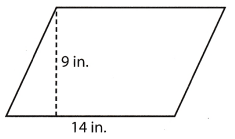(A) 42 square inches (B) 63 square inches (C) 126 square inches (D) 252 square inches Answer: (C) 126 square inches Explanation: Determine the area of the parallelogram. A = bh. formula for the area of a parallelogram A = 14 in × 9 in substitute for the given values A = 126 square inches area of the parallelogram The area of the parallelogram is 126 square inches. Question 9. Which shows the integers in order from least to greatest? (A) 17, 3, 2, -5, -16 (B) -5, 2, 3, -16, 17 (C) -16, -5, 2, 3, 17 (D) 17, -16, 3, 2, -5 Answer: (C) -16, -5, 2, 3, 17 Explanation: Integers are arranged from least to greatest starting from the negative number going to positive numbers. Integers arranged from least to greatest is 16, -5, 2, 3, 17. Gridded Response Question 10. What is the debt-to-income ratio for the data below written as a percent? Monthly income:$2,750
Car loan payment: $375 Credit card payment:$120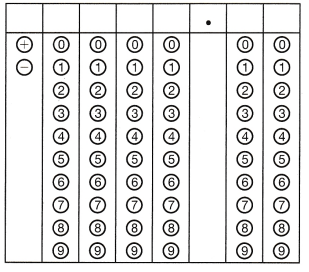Determine the debt-to-income ratio.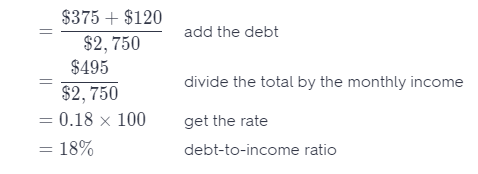The debt-to-income ratio is 18%.

Question 11.
Andrea is attending a university where the cost for one year is $13,500. She has a scholarship worth$5,000 and a grant worth $1,700. She earns$40 a day at her job. How many days does she need to work to pay for 30% of the remaining amount?= $13,500 – ($5,000 + $1, 700) add the debt =$13,500 – $6,700 subtract the total debt from the monthly income =$6,800 remaining amount
30% of $6,800 =$2,040 percentage of the remaining amount she needs to pay
$2,040 ÷$40 = 51 days number of days she needs to work
Shea has an annual salary of $29,640. He works 40 hours a week, 52 weeks a year. How much does Shea earn per hour?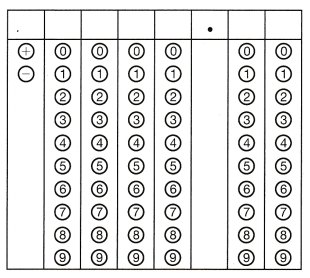Answer: Determine the amount she worked in an hour.$29,640 ÷ 52 weeks = $570 amount earned per week$570 ÷ 40 hours = $14.25 amount earned per hour Shea earns$14.25 per hour.